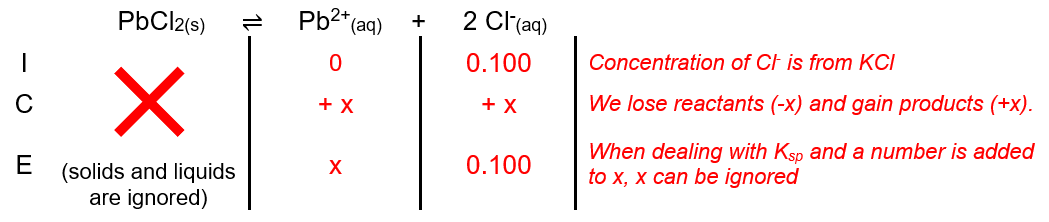# Problem: Calculate the molar solubility of PbCl 2 (Ksp = 1.6 x 10-5 at 25 degrees) in a 0.100 M solution of KCl.

###### FREE Expert Solution

For this problem, we’re being asked to calculate the solubility (in mol/L) of PbCl2(s) in 0.100 M KCl(aq). Since the compounds are ionic compounds, they form ions when dissociating in water. The dissociation of PbCl2 and KCl in water are as follows:

The chloride ion, Cl-, has a charge of –1. Pb is a transition metal.

Since ionic compounds criss-cross charges which becomes subscripts:

• Subscript 2 of Cl must have come from Pb → so the charge of Pb is +2 → Pb2+

• Subscript 1 of Pb must have come from the -1 charge of Cl →  Cl-

PbCl2(s)  Pb2+(aq)2 Cl(aq)

The chloride ion, Cl-, has a charge of –1. Potassium is in Group 1A so it’s charge is +1:

KCl(aq)  K+(aq) + Cl(aq)

Notice that there is a common ion present, Cl. The common ion effect states that the solubility of a salt is lower in the presence of a common ion.

We can construct an ICE table for the dissociation of PbCl2. Remember that solids are ignored in the ICE table.84% (7 ratings)###### Problem Details

Calculate the molar solubility of PbCl (Ksp = 1.6 x 10-5 at 25 degrees) in a 0.100 M solution of KCl.